# KSEEB Solutions for Class 7 Maths Chapter 2 Fractions and Decimals Ex 2.2

Students can Download Chapter 2 Fractions and Decimals Ex 2.2, Question and Answers, Notes Pdf, KSEEB Solutions for Class 7 Maths, Karnataka State Board Solutions help you to revise complete Syllabus and score more marks in your examinations.

## Karnataka State Syllabus Class 7 Maths Chapter 2 Fractions and Decimals Ex 2.2

Question 1.
Which of the drawings (a) to (d) show :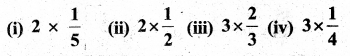Solution: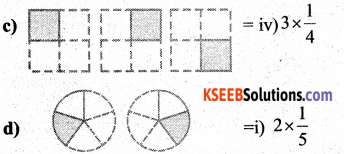Question 2.
Some pictures (a) to (c) are given below. Tell which of them show :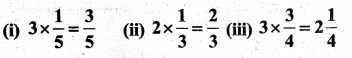Solution: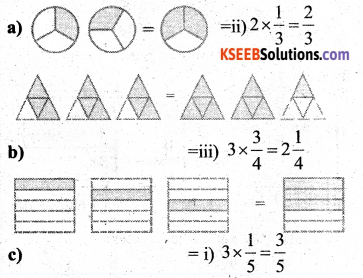Question 3.
Multiply and reduce to lowest form and convert into a mixed fraction :
Solution: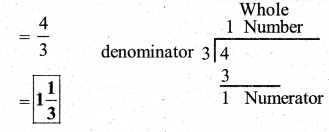iii)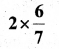Solution: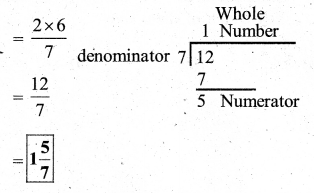iv)Solution: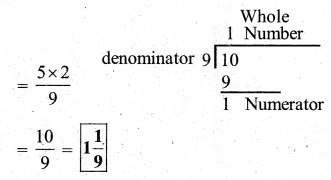v)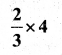Solution:vi)Solution: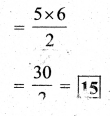vii)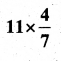Solution:viii)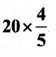Solution:ix)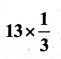Solution: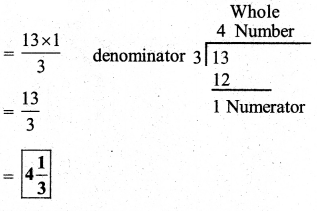Question 4.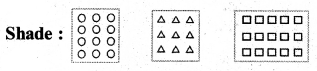i) $$\frac{1}{2}$$ of the circles in box (a)
Solution: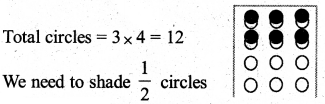ii) $$\frac{2}{3}$$ of the triangle in box(b)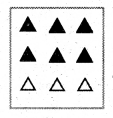Solution:
Total triangles = 3 × 3 = 9
we need to shade $$\frac{2}{3}$$ triangles number of circles to shade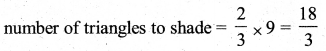iii) $$\frac{3}{5}$$ of the squares in box (c).
Solution: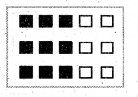Total squares = 5 × 3 = 15
we need to shade $$\frac{3}{5}$$ squares.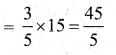(∴ So shading squares = 9)

Question 5.
Find:
a)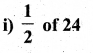Solution: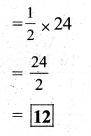ii)Solution: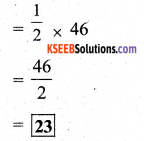b)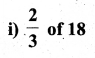Solution: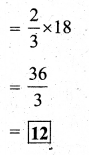ii)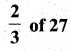Solution:c)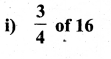Solution:ii)Solution: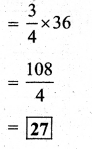d)Solution:ii)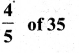Solution:Question 6.
Multiply and express a mixed fraction:
a)Solution: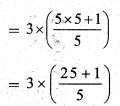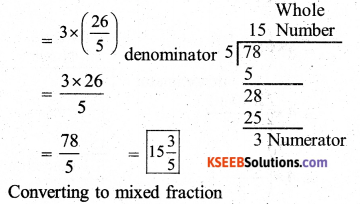b)Solution: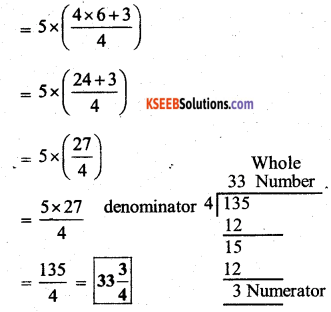c)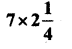Solution:d)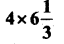Solution:e)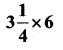Solution: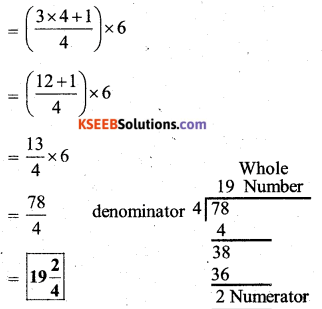f)Solution: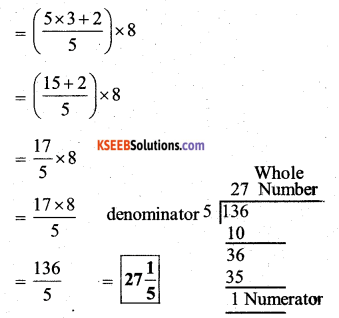Question 7.
Find:
a)Solution: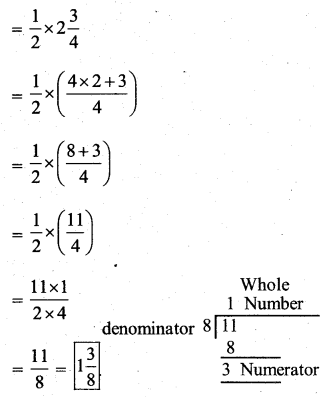ii)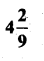Solution: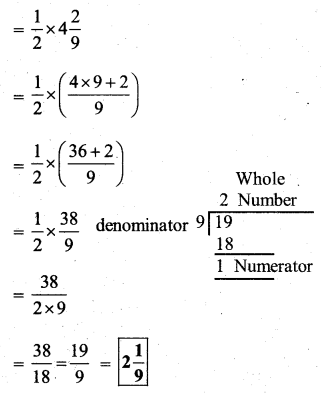b)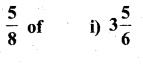Solution: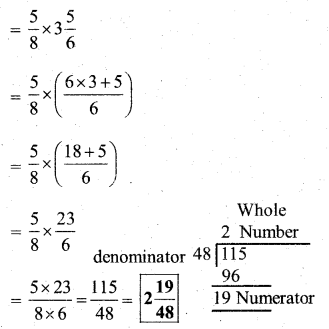ii)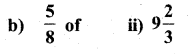Solution: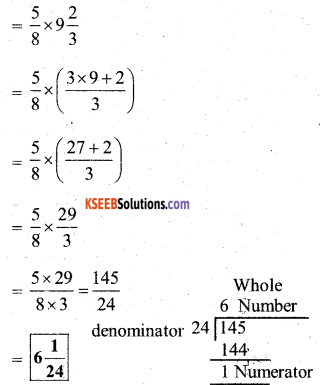Question 8.
Vidya and Pratap went for a picnic. Their mother gave them a water bottle that contained 5 litres of water. Vidya consumed $$\frac{2}{5}$$ of the water. Pratap consumed the remaining water,
i) How much water did Vidya drink ?
Solution:
Total water = 5 liters
Given that,
Vidya drank $$\frac{2}{5}$$ of the water.
∴ Water Vidya drank = $$\frac{2}{5}$$ × Total water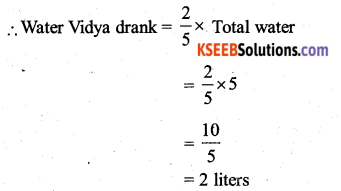(∴ Vidya drank 2 liters of water)

(ii) What fraction of the quantity of water did Pratap drink ?
Solution:
Now,
Water pratap drink = Total water – water vidhya drink
= 5 – 2
= 3 liters
∴ Pratap drink 3 liters of water
fraction of total water pratap drink =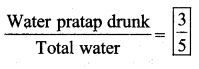error: Content is protected !!